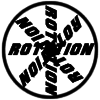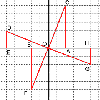#### You may also like### 8 Methods for Three by One

This problem in geometry has been solved in no less than EIGHT ways by a pair of students. How would you solve it? How many of their solutions can you follow? How are they the same or different? Which do you like best?### Rots and Refs

Follow hints using a little coordinate geometry, plane geometry and trig to see how matrices are used to work on transformations of the plane.### The Matrix

Investigate the transfomations of the plane given by the 2 by 2 matrices with entries taking all combinations of values 0. -1 and +1.

# Reflect Again

##### Age 16 to 18Challenge Level

Andrei Lazanu proved the double angle formulae illustrated in the diagram:

The diagram starts from a right angled triangle, of sides $2t$ and 2 and where consequently $\tan\theta = t$. In this triangle, a line making an angle $\theta$ with the hypotenuse is drawn. This way, an isosceles triangle is formed, and $2\theta$ is the angle exterior to this isosceles triangle. Let the sides DA and DB of this isosceles triangle be $x$\ units. Then the length of DC must be $2-x$ units. Using Pythagoras' Theorem for triangle ADC we find $x$. $$x^2=(2t)^2+(2-x)^2.$$ Hence $x=1+t^2$ and so the length of side DC is $2-(1+t^2)=1-t^2$.

The formulae for the sine, cosine and tangent of $2\theta$ in terms of $t$, where $t=\tan \theta$, follow directly from the ratios of the sides of the right angled triangle ADC and we get $$\tan2\theta = {2t\over {1-t^2}},\quad \sin2\theta = {2t\over {1+t^2}},\quad \cos2\theta = {{1-t^2}\over {1+t^2}}$$
Rots and Refs provides a proof of the translation formula .

Finally, to find the combined transformation you have to multiply the transformation matricies to get (using the double angle formulae to simplify):

$$T= \left( \begin{array}{cc} cos 2(\phi-\theta) & -sin2(\phi-\theta)\\ sin2(\phi-\theta & \cos2(\phi-\theta) \end{array} \right)$$
Which is a rotation. If the angles are equal the matrix would be:

$$T= \left( \begin{array}{cc} 1 & 0\\ 0 & 1 \end{array} \right)$$
So the two reflections combined would leave all points unchanged.